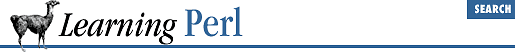home | O'Reilly's CD bookshelfs | FreeBSD | Linux | Cisco | Cisco Exam

### 8.3 Return Values

A subroutine is always part of some expression. The value of the subroutine invocation is called the return value . The return value of a subroutine is the value of the return statement or of the last expression evaluated in the subroutine.

For example, let's define this subroutine:

```sub sum_of_a_and_b {
return \$a + \$b;
}```

The last expression evaluated in the body of this subroutine (in fact, the only expression evaluated) is the sum of ``` \$a``` and ``` \$b``` , so the sum of ``` \$a``` and ``` \$b``` will be the return value. Here's that in action:

```\$a = 3; \$b = 4;
\$c = sum_of_a_and_b();     # \$c gets 7
\$d = 3 * sum_of_a_and_b(); # \$d gets 21```

A subroutine can also return a list of values when evaluated in a list context. Consider this subroutine and invocation:

```sub list_of_a_and_b {
return(\$a,\$b);
}
\$a = 5; \$b = 6;
@c = list_of_a_and_b(); # @c gets (5,6)```

The last expression evaluated really means the last expression evaluated, rather than the last expression defined in the body of the subroutine. For example, this subroutine returns ``` \$a``` if ``` \$a``` ``` >``` ``` 0``` ; otherwise it returns ``` \$b``` :

```sub gimme_a_or_b {
if (\$a > 0) {
print "choosing a (\$a)\n";
returns \$a;
} else {
print "choosing b (\$b)\n";
returns \$b;
}
}```

These are all rather trivial examples. It gets better when we can pass values that are different for each invocation into a subroutine instead of relying on global variables. In fact, that's coming right up.8.2 Invoking a User Function8.4 Arguments